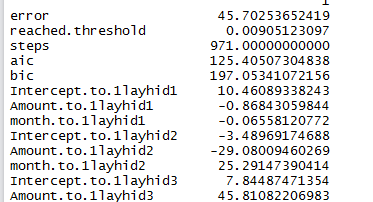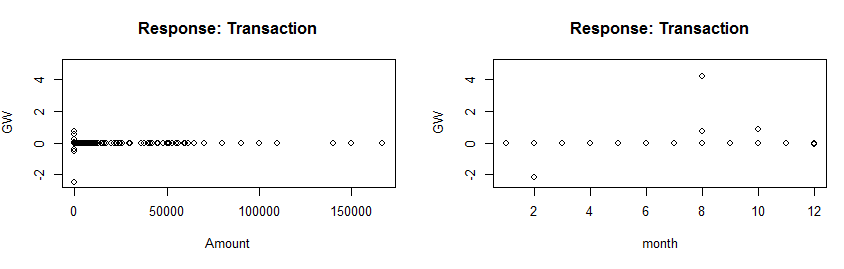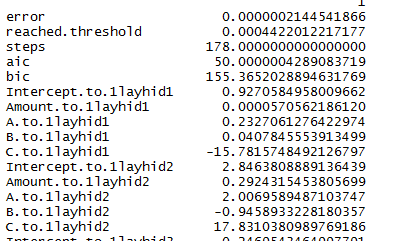## Tuesday, May 27, 2014

### The Problem posted previously neural not respond I tried to find the problem based on actual data-

Now First I tried to run the logic on existing set of data -

`````` library("neuralnet")
setClass("myDate")
#Replace the comma from Amount
data\$Amount <- as.numeric(gsub(",", "", gsub("", "", data\$Amount)))
#Change Dr.(1) and Cr.(0)
data\$Transaction <- as.numeric(data\$Transaction=="Dr.")
#Split Date in Day Month & year
data\$Transaction.Date <- as.Date(data\$Transaction.Date, format="%d/%m/%Y")
month = as.numeric(format(data\$Transaction.Date, format = "%m"))
year = as.numeric(format(data\$Transaction.Date, format = "%Y"))
``````

And the data looks like-

For this data I already provided different plots based on different perm comp here Previous

And as we know we get a huge Error rate after running the neural network algorithm -

`````` output <- neuralnet(Transaction ~ Amount+month,data,hidden = 4,threshold = 0.01,linear.output=FALSE, likelihood=TRUE)
print(output\$result)
plot(output,rep = "best")
``````I am going to present an another way of visualizing the result.
Visualize the result from using generalized Weights
gwplot uses  the calculated generalized weight provided by nn\$generalized.weights

`````` out <- cbind(output\$covariate,output\$net.result[])
dimnames(out) <- list(NULL, c("Amount","Month","nn-output"))
``````

`````` #Plotting Generalized weight
#The distribution of the generalized weights suggests that the covariate Amount
#has no effect on the case-control status since all generalized weights are nearly zero
par(mfrow=c(2,2))
gwplot(output,selected.covariate="Amount", min=-2.5, max=5)
gwplot(output,selected.covariate="month", min=-2.5, max=5)
``````The distribution of the generalized weights suggests that the covariate Amount has no effect on the case-control status since all generalized weights are nearly zero

### I Added few features in my Data Set with some sense and again did all these steps

`````` library("neuralnet")
data_new <- data;
data_new[c("A","B","C")] <- NA
data_new\$A <- sample(1:10,nrow(data_new),replace = TRUE)
data_new\$B <- sample(22:30,nrow(data_new),replace = TRUE)
data_new\$C <- as.numeric(data_new\$Transaction =="1")
``````

And after running rest of the code,

`````` plot(data_new\$Amount, data_new\$A+data_new\$B+data_new\$C, main="Transaction vs Amount",
xlab="Amount", ylab="A+B+C", pch=1, col="red")
output_new <- neuralnet(Transaction ~ Amount+A+B+C,data_new,hidden = 4,threshold = 0.01,linear.output=FALSE, likelihood=TRUE)
print(output_new\$result)
plot(output_new,rep = "best")
#How well my Data Fits Here
out_new <- cbind(output_new\$covariate,output_new\$net.result[])
dimnames(out_new) <- list(NULL, c("Amount","A","B","C","neural-output"))
par(mfrow=c(2,2))
#two covariates A and C have a nonlinear effect since
#the variance of their generalized weights is overall greater than one
gwplot(output_new,selected.covariate="Amount", min=-2.5, max=5)
gwplot(output_new,selected.covariate="A", min=-2.5, max=5)
gwplot(output_new,selected.covariate="B", min=-2.5, max=5)
gwplot(output_new,selected.covariate="C", min=-2.5, max=5)
``````

I got an excellent Error rate as well as distribution of the generalized weights were goodtwo covariates A and C have a nonlinear effect since  the variance of their generalized weights is overall greater than one